888.815.0604
Home / Resources / Microsoft Office / Excel / Using FV Function

# Using the FV Function

In this article, we will delve a little deeper into how to use the FV Function in Microsoft Excel.

For instructor delivered MS Excel training in Chicago and Los Angeles call us on 888.815.0604..

Another commonly used financial function is the FV (future value) function. This function returns the future value of a series of periodic payments to an investment at a fixed interest rate. For example, if you put \$5,000 a year into an investment that yields 3.5% annual interest, the FV function can tell you how much your investment will be worth after a given period.

To use this function, first select the cell in which you would like the results to be displayed. For this example, click cell D2. Next, click Formulas → Financial → FV:This action will open the Function Arguments dialog for the FV function:The top portion of this dialog contains a number of arguments that you can use to tailor this function. The arguments in bold are required in order for this function to operate. Arguments not in bold are optional: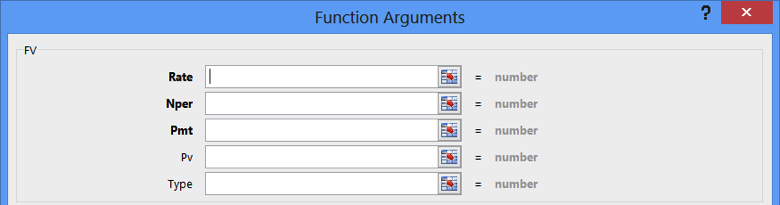The Rate argument is the interest rate at which you expect to earn on the investment value. In the current workbook, interest rates are listed in column A, so with the Rate field selected, click cell A2: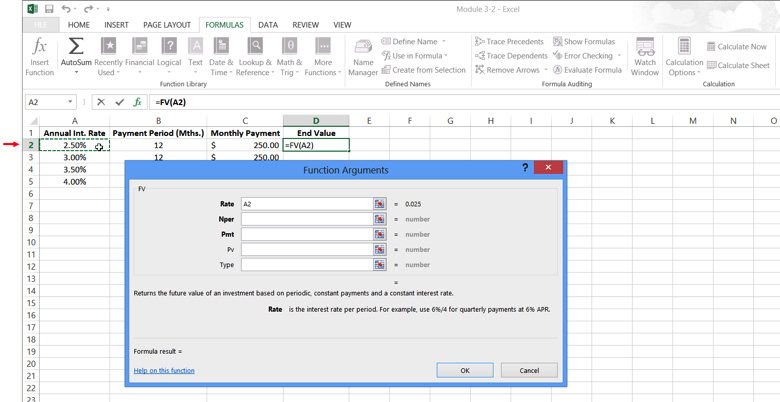You need to account for the fact that the values in column A are annual interest rates, so you need to divide this data by 12. In the Rate argument field, type /12 after A2: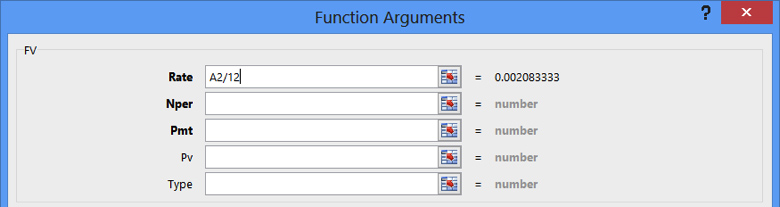Next, the Nper argument is the number of payment periods over which contributions will be made to the investment. In the sample workbook, all payment periods are stored in column B, so with the Nper field selected, click B2: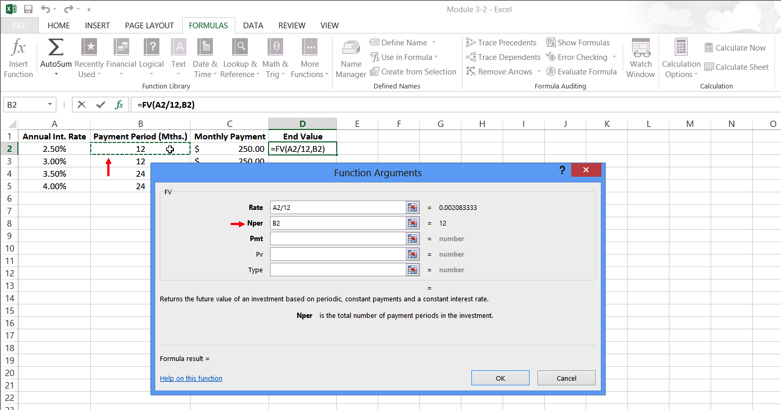The Pmt argument is used to define the payment that you will make in each period. In this workbook, payment amounts are stored in column C. With the Pmt field selected, type -C2: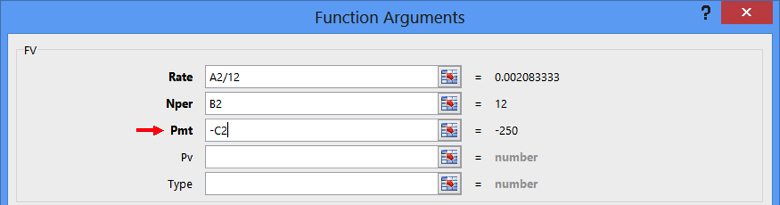The remaining arguments left in this function are optional.

• The Pv argument is use to set a lump sum that you can begin with. (By default, this argument is 0.)
• The Type argument will specify if the payment is made at the beginning or end of the payment period: enter 1 for payments at the beginning of the payment period and 0 for payments at the end of the payment period. (By default, this argument is also 0.)

The Function Arguments dialog will now look like this: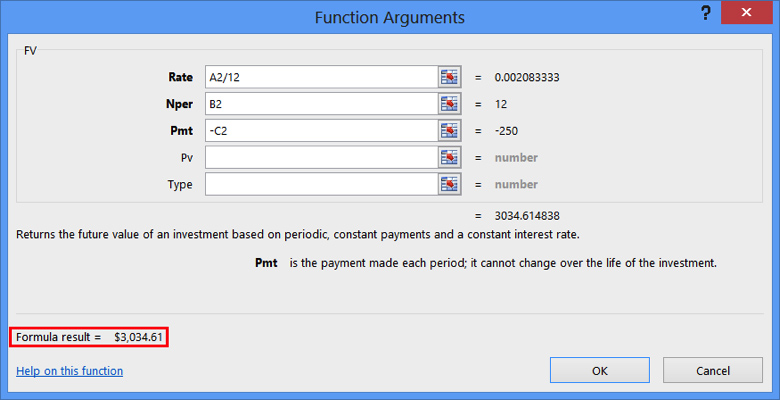The result of the function indicates the end value of this investment will be \$3,034.61. Click OK to insert this function: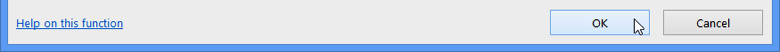The result of the function will now be displayed in cell D2 of the current worksheet: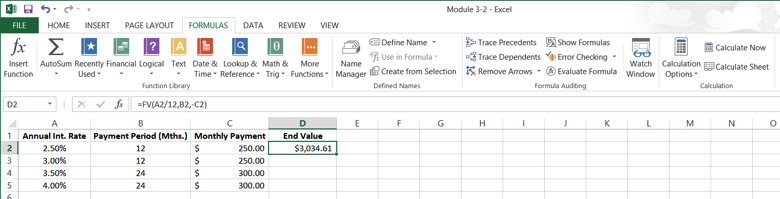Using AutoFill, apply this function to cells D3-D5: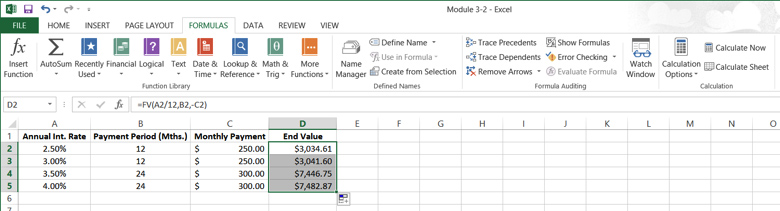When using the FV function, you do not need to use the Function Arguments dialog. You can type the function directly into the formula bar using “FV” as a prefix: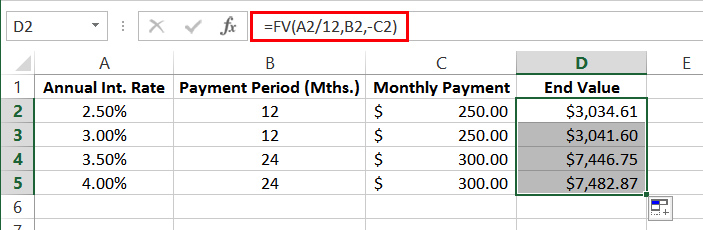## Class based Microsoft Excel available in Chicago and LA

Join our 1-day Excel workshop. We run classes for every skill level each month. Check out real student testimonials Excel class reviews.

## Excel courses